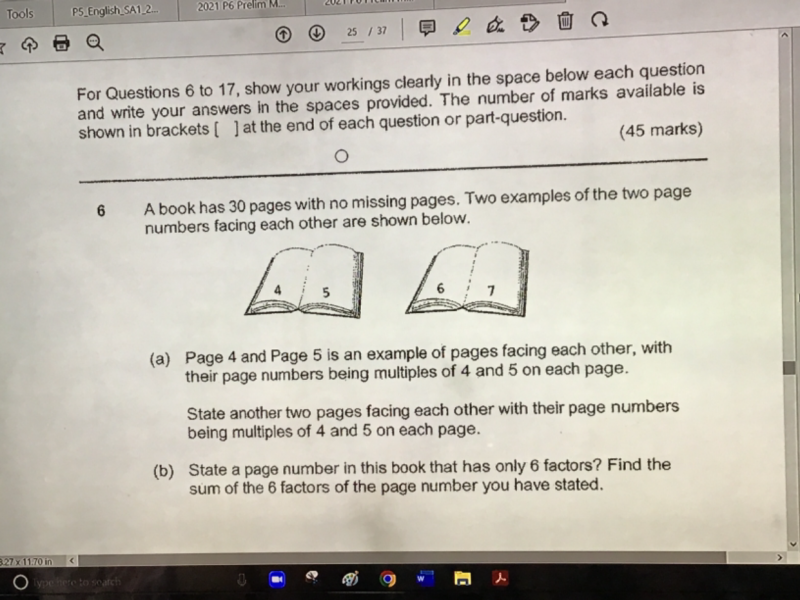# QuestionCan the answer for (b) be 18, 39? Thanks

(a) 24, 25

(b) This question has many answers.   I would think they have asked for 8 factors because there is only ONE answer for that.  Page 24, Sum = 60

6 factors are as follows and any answer is correct.

Page 12  —> 1 + 12 + 2 + 6 + 3 + 4 = 28

Page 18  —> 1 + 18 + 2 + 9 + 3 + 6 = 39

Page 20 —> 1 + 20 + 2 + 10 + 4 + 5 = 42

Page 28 —> 1 + 28 + 2 + 14 + 4 +7 = 56

Page 30 —> 1 + 30 +2 + 15 + 3 + 10 = 61